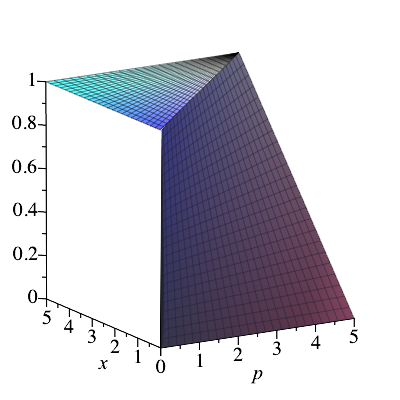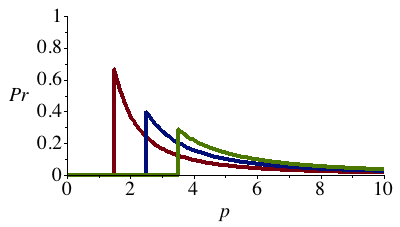## Friday, May 9, 2014

### Zabell: "R. A. Fisher and the Fiducial Argument" (1992)

Chapter 3.3 of Fisher's 1956 book is dedicated to his so-called "Fiducial Argument."

I was extremely confused by his presentation and looked around for some secondary literature. This brought me to this wonderful paper by Sandy Zabell, which explains how Fisher's ideas about fiducial inference were indeed quite confused and changed a lot over time. It also explains the core of his argument better than he did himself (to my mind, at least).

As I now understand Fisher's argument, this is the idea: When you have a statistical model with a flat prior, the posterior probability of a specific parameter setting is proportional to the likelihood of the data under that parameter setting,

Pr(p | x) ∝ Pr(x | p)

However, for many unbounded parameter spaces, the likelihood Pr(X = x | p) does not have a finite integral when considered as a function of p. In such cases, a straightforward use of Bayesian inference with a flat prior is not an option.

But, Fisher says, consider instead the cumulative likelihood Pr(X < x | p). This is a function of x which always lies between 0 and 1, and it is 0 at negative infinity and 1 at positive infinity.Pr(X < x | p) for uniform distributions with right end-points p = 3, p = 5, and p = 7.

The trick now is to view this cumulative likelihood as a function of p instead of a function of x. In many but not all cases, this function will be 1 when the parameter is at negative infinity and 0 when it is at positive infinity.Cumulative likelihood at x = 1.5, x = 2.5, and p = 3.5 as a function of the parameter.

For instance, if p is the mean of a normal distribution, the cumulative likelihood Pr(X < x | p) decreases in this way. The reason is that the upper bound x stays where it is, while the expected value of the variable increases.Uniform likelihoods Pr(X < x | p) with varying right end-points p.

In such cases, we can thus interpret the cumulative likelihood as the complement of a CDF for the parameter,
G(p) = 1 – Pr(X < x | p).
If this function G is differentiable, we can further interpret G' as a PDF for the parameter p given observation x.

As an example, suppose (as on the pictures) that a number X is drawn from a uniform distribution on the interval [0, p]. The cumulative likelihood is then
Pr(X < x | p) = x/p    (0 < x < p),
and 0 and 1 below and above the interval, respectively.Fiducial PDFs given the observations x = 1.5, x = 2.5, and x = 3.5.

Considering the complement of this function, 1 – x/p, as a CDF for the parameter p, we can differentiate it in order to get the density
G'(p) = x/p2    (x < p),
when p > x and 0 otherwise. We have thus obtained a posterior probability distribution for the parameter without assuming anything about the prior.

It should be noted that
• This method does not always work; consider for instance the case in which the cumulative likelihood oscillates between a unimodal normal and a bimodal normal distribution as the parameters runs along the real number line.
• The method can also give inconsistent results; for instance, the fiducial distribution of X2 is, as far as I understand, not necessarily the distribution you would get by finding the fiducial distribution of X and then deriving a distribution for X2.
• The method has no single, natural extension to the multi-parameter case, and there are some serious obstacles to constructing such an extension.
It is also interesting that in the example above, the fiducial distribution corresponds to the posterior you get if you assume the improper prior 1/p. It can thus not be rationalized as a posterior inference using only ordinary probability distributions, but it can if we allow ourselves crazy, unnormalizable ones.